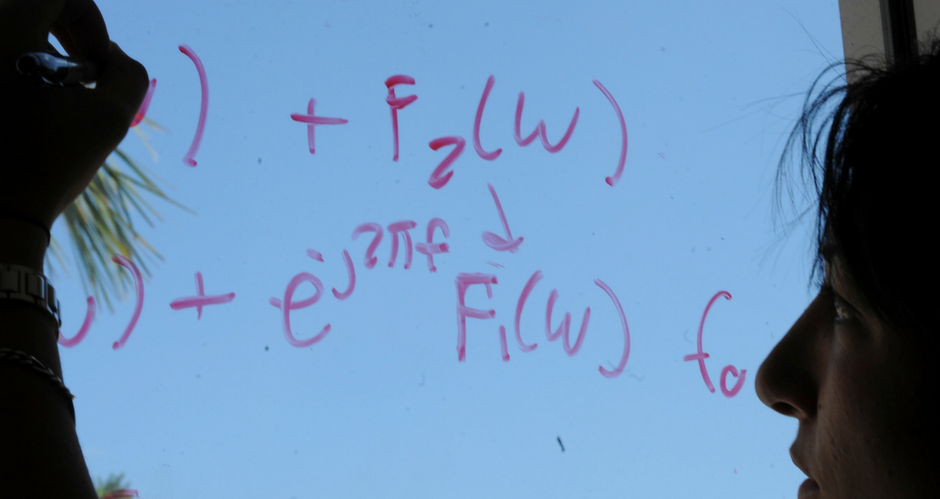## Mathematics and System Engineering Faculty Publications

Article

#### Publication Title

Electronic Journal of Differential Equations

#### Abstract

Using the theory of fixed point index, this paper discusses the existence of at least one positive solution and the existence of multiple positive solutions for the singular three-point boundary value problem: y″(t) + a(t)f(t, y(t), y′(t)) = 0, 0 < t < 1, y′(0) = 0, y(1) = αy(η), where 0 < α < 1, 0 < η < 1, and f may be singular at y = 0 and y′ = 0. .

2008

COinS